Home Practice
For learners and parents For teachers and schools
Textbooks
Full catalogue
Pricing SupportLog in

We think you are located in United States. Is this correct?

# End of chapter exercises

## End of chapter exercises

Textbook Exercise 8.7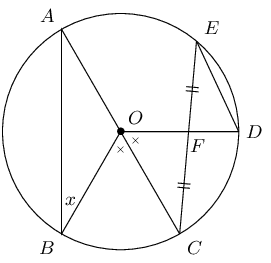$$AOC$$ is a diameter of the circle with centre $$O$$. $$F$$ is the mid-point of chord $$EC$$. $$B\hat{O}C = C\hat{O}D$$ and $$\hat{B} = x$$. Express the following angles in terms of $$x$$, stating reasons:

$$\hat{A}$$
$\begin{array}{rll} \hat{A} &= x & (\text{radius } OA = OB) \end{array}$
$$C\hat{O}D$$
$\begin{array}{rll} C\hat{O}B &= \text{2}x & (\text{angles at centre and on circumference})\\ &= C\hat{O}D & \text{(given)}\\ &= \text{2} x & \end{array}$
$$\hat{D}$$
$\begin{array}{rll} \hat{E} &= \frac{1}{2} C\hat{O}D & \\ &= x & (\angle\text{'s at centre and circumference})\\ \therefore\hat{D} &= \text{90}\text{°} - x & (\text{sum } \angle {s } \triangle = \text{180}\text{°}) \end{array}$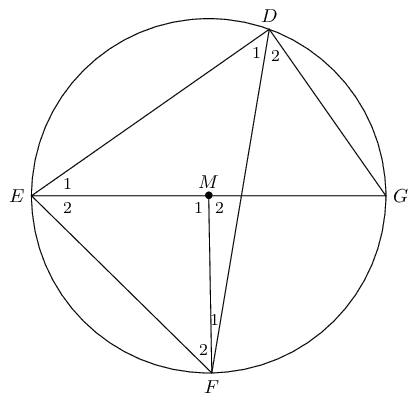$$D$$, $$E$$, $$F$$ and $$G$$ are points on circle with centre $$M$$. $$\hat{F}_1 = \text{7}\text{°}$$ and $$\hat{D}_2 = \text{51}\text{°}$$. Determine the sizes of the following angles, stating reasons:

$$\hat{M}_1$$
$\begin{array}{rll} \hat{M_2} &= 2 \times \text{51}\text{°} & (\angle \text{ at centre } = \text{2}\times \text{circumference})\\ \therefore \hat{M_2} &= \text{102}\text{°} & \\ \hat{M_1} &= \text{180}\text{°} - \text{102}\text{°} & (\angle \text{ on str.line })\\ \therefore \hat{M_1} &= \text{78}\text{°} & \end{array}$
$$\hat{D}_1$$
$\begin{array}{rll} \hat{D_1} &= \frac{1}{2} \times \text{78}\text{°} & (\angle \text{ at centre } = \text{2} \times \text{ circumference})\\ \therefore \hat{D_1} &= \text{39}\text{°} & \end{array}$
$$\hat{F}_2$$
$\begin{array}{rll} \hat{F_2} + \hat{E_2} &= \text{102}\text{°} & (\text{exterior } \angle \triangle) \\ \hat{F_2} &= \hat{E_2} &(ME = MF)\\ &= \text{51}\text{°} & \end{array}$
$$\hat{G}$$
$\begin{array}{rll} \hat{G} &= E\hat{F}D & (\angle \text{s on same chord})\\ &= \text{58}\text{°} & \end{array}$
$$\hat{E}_1$$
$\begin{array}{rll} \hat{E_1} &= \text{180}\text{°} - \text{90}\text{°} - \hat{G} & \\ &= \text{180}\text{°} - \text{90}\text{°} - \text{58}\text{°}& \\ \therefore \hat{E_1} &= \text{32}\text{°} & \end{array}$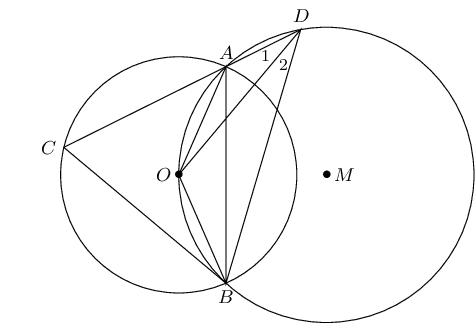$$O$$ is a point on the circle with centre $$M$$. $$O$$ is also the centre of a second circle. $$DA$$ cuts the smaller circle at $$C$$ and $$\hat{D}_1 = x$$. Express the following angles in terms of $$x$$, stating reasons:

$$\hat{D}_2$$
$\begin{array}{rll} \hat{D_2} &= \hat{D_1} &(\angle\text{s on same chord} OA = OB) \\ &= x & \end{array}$
$$O\hat{A}B$$
$\begin{array}{rll} O\hat{A}B &= x &(\angle\text{'s on same chord}) \end{array}$
$$O\hat{B}A$$
$\begin{array}{rll} O\hat{B}A &= x &(\text{equal radii} OA=OB) \end{array}$
$$A\hat{O}B$$
$\begin{array}{rll} A\hat{O}B &= \text{180}\text{°} - \text{2}x &(\text{sum } \angle\text{s } \triangle = \text{180}\text{°}) \end{array}$
$$\hat{C}$$
$\begin{array}{rll} \hat{C} &= \text{90}\text{°} - x &(\angle\text{'s on same chord}) \end{array}$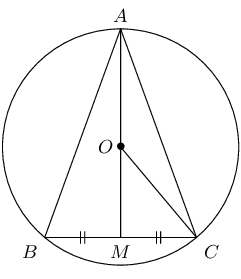$$O$$ is the centre of the circle with radius $$\text{5}$$ $$\text{cm}$$ and chord $$BC = \text{8}\text{ cm}$$. Calculate the lengths of:

$$OM$$
$\begin{array}{rll} \text{In } OMC, \quad OC^2 &= OM^2 + MC^2 &({Pythagoras})\\ 5^2 &= OM^2 + 4^2 & \\ \therefore OM &= \text{3}\text{ cm} & \end{array}$
$$AM$$
$\begin{array}{rll} AM &= \text{5} + \text{3} & \\ \therefore AM &= \text{8}\text{ cm} & \\ \end{array}$
$$AB$$
$\begin{array}{rll} \text{In } ABM, \quad AB^2 &= BM^2 + AM^2 &({Pythagoras}) \\ AB^2 &= 8^2 + 4^2 & \\ AB &= \sqrt{80} & \\ \therefore AB &= 4\sqrt{5}\text{cm} & \end{array}$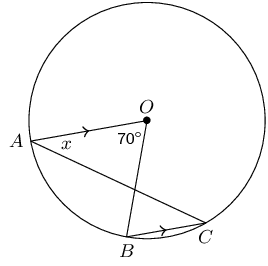$$AO \parallel CB$$ in circle with centre $$O$$. $$A\hat{O}B = \text{70}\text{°}$$ and $$O\hat{A}C = x$$. Calculate the value of $$x$$, giving reasons.

$\begin{array}{rll} \hat{C} &= \frac{1}{2} A\hat{O}B & (\angle\text{s at centre = twice circumference})\\ &= \text{35}\text{°} & \\ \therefore x &= \text{35}\text{°} &(\text{alt. } \angle\text{'s, } AO \parallel BC) \end{array}$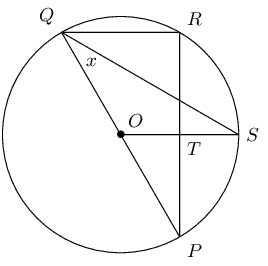$$PQ$$ is a diameter of the circle with centre $$O$$. $$SQ$$ bisects $$P\hat{Q}R$$ and $$P\hat{Q}S = x$$.

Write down two other angles that are also equal to $$x$$.

$\begin{array}{rll} R\hat{Q}S &= x & \\ Q\hat{S}O &= x & \end{array}$

Calculate $$P\hat{O}S$$ in terms of $$x$$, giving reasons.

$\begin{array}{rll} P\hat{O}S &= \text{2}\times P\hat{Q}S&(\angle \text{'s at centre and circumference on same chord}) \\ &= \text{2}x & \end{array}$

Prove that $$OS$$ is a perpendicular bisector of $$PR$$.

$\begin{array}{rll} x_1 &= x_2 & (\text{proven}) \\ \therefore QR &\parallel OS & \\ \therefore \hat{R} &= R\hat{T}S & (\text{alt. } \angle \text{'s, } QR \parallel OS)\\ &= \text{90}\text{°} &(\hat{R} = \angle \text{ in semi-circle})\\ \therefore PT &= TR & \\ \therefore OS &\text{ is perpendicular bisector of } PR & \end{array}$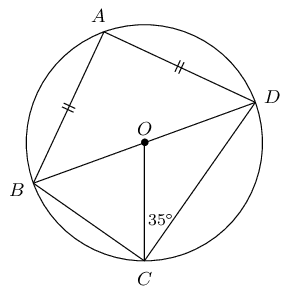$$B\hat{O}D$$ is a diameter of the circle with centre $$O$$. $$AB = AD$$ and $$O\hat{C}D = \text{35}\text{°}$$. Calculate the value of the following angles, giving reasons:

$$O\hat{D}C$$
$\begin{array}{rll} O\hat{D}C &= \text{35}\text{°}&(\text{radii } OC=OD) \end{array}$
$$C\hat{O}D$$
$\begin{array}{rll} C\hat{O}D &= \text{180}\text{°} - \text{70}\text{°} &(\text{sum} \angle\text{'s } \triangle = \text{180}\text{°}) \\ &=\text{110}\text{°} & \end{array}$
$$C\hat{B}D$$
$\begin{array}{rll} C\hat{B}D &= \frac{1}{2} C\hat{O}D&(\angle\text{'s on } CD)\\ &= \text{55}\text{°} & \end{array}$
$$B\hat{A}D$$
$\begin{array}{rll} B\hat{A}D &= \text{90}\text{°} & (\angle\text{ in semi-circle}) \end{array}$
$$A\hat{D}B$$
$\begin{array}{rll} A\hat{D}B &= \frac{\text{180}\text{°}-\text{90}\text{°}}{2}&(\text{sum } \angle\text{'s in } \triangle = \text{180}\text{°})\\ &= \text{45}\text{°} & \end{array}$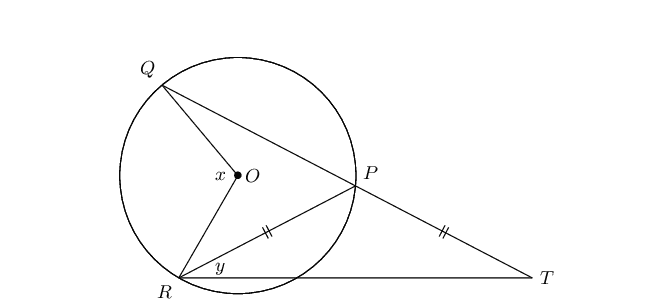$$QP$$ in the circle with centre $$O$$ is protracted to $$T$$ so that $$PR = PT$$. Express $$y$$ in terms of $$x$$.

$\begin{array}{rll} T &= y &(PT = PR)\\ \therefore Q\hat{P}R &= \text{2}y &(\text{ext. } \angle \triangle = \text{ sum interior } \angle\text{'s})\\ \therefore x &= \text{2}(\text{2}y)& (\angle\text{'s at centre and circumference on } QR)\\ x &= 4y & \end{array}$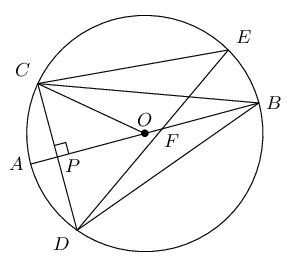$$O$$ is the centre of the circle with diameter $$AB$$. $$CD \perp AB$$ at $$P$$ and chord $$DE$$ cuts $$AB$$ at $$F$$. Prove that:

$$C\hat{B}P = D\hat{P}B$$
$\begin{array}{rll} \text{In } \triangle CBP &\text{ and } \triangle DBP\text{:}& \\ CP &= DP& (OP \perp CD)\\ C\hat{P}B &= D\hat{P}B = \text{90}\text{°} & (\text{given}) \\ BP &= BP & (\text{common})\\ \therefore \triangle CBP &\equiv DBP &(\text{SAS} ) \end{array}\\$
$$C\hat{E}D = 2 C\hat{B}A$$
$\begin{array}{rll} C\hat{E}D &= C\hat{B}D& (\angle\text{'s on chord } CD)\\ \text{But } C\hat{B}A &= D\hat{B}A & (\triangle CBP \equiv \triangle DBP)\\ \therefore C\hat{E}D &= 2 C\hat{B}A \end{array}$
$$A\hat{B}D = \frac{1}{2} C\hat{O}A$$
$\begin{array}{rll} D\hat{B}A &= C\hat{B}A & (\triangle CBP \equiv \triangle DBP)\\ C\hat{B}A &= \frac{1}{2} C\hat{O}A & (\angle\text{'s at centre and circumference on arc } AC)\\ \therefore A\hat{B}D &= \frac{1}{2} C\hat{O}A & \end{array}$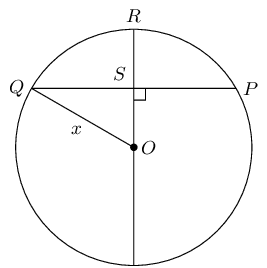In the circle with centre $$O$$, $$OR \perp QP$$, $$PQ = \text{30}\text{ mm}$$ and $$RS = \text{9}\text{ mm}$$. Determine the length of $$OQ$$.

$\begin{array}{rll} \text{In } &\triangle QOS, & \\ QO^2 &= OS^2 + QS^2 & ( \text{Pythagoras }) \\ x^2 &= (x-9)^2 + 15^2 & \\ x^2 &= x^2 - 18x + 81 + 225 & \\ \therefore 18x &= 306 & \\ \therefore x &= 17 & \end{array}$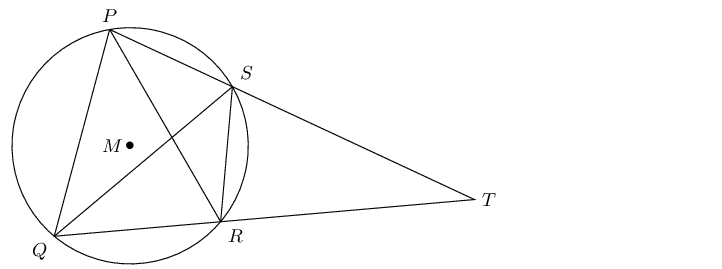$$P$$, $$Q$$, $$R$$ and $$S$$ are points on the circle with centre $$M$$. $$PS$$ and $$QR$$ are extended and meet at $$T$$. $$PQ = PR$$ and $$P\hat{Q}R = \text{70}\text{°}$$.

Determine, stating reasons, three more angles equal to $$\text{70}\text{°}$$.

$$Q\hat{R}P,Q\hat{S}P,R\hat{S}T$$

If $$Q\hat{P}S = \text{80}\text{°}$$, calculate $$S\hat{R}T$$, $$S\hat{T}R$$ and $$P\hat{Q}S$$.

$\begin{array}{rll} \text{In } &\triangle QOS, & \\ S\hat{R}T &= \text{80}\text{°} & ( \text{ext. angle cyclic quad. }) \\ S\hat{T}R &= \text{180}\text{°}- \text{80}\text{°}- \text{30}\text{°} & (\angle \text{s sum of } \triangle) \\ \therefore S\hat{T}R = \text{30}\text{°} & \\ In \triangle PQS, \quad P\hat{Q}S &= \text{180}\text{°}- \text{80}\text{°}- \text{30}\text{°} & (\angle \text{s sum of } \triangle) \\ \therefore P\hat{Q}S &= \text{30}\text{°} & \end{array}$

Explain why $$PQ$$ is a tangent to the circle $$QST$$ at point $$Q$$.

$$P\hat{Q}S = Q\hat{T}S = \text{30}\text{°}$$, therefore $$PQ$$ is a tangent to the circle through $$QST$$, (angle between line and chord equals angle in alt. seg.)

Determine $$P\hat{M}Q$$.

$\begin{array}{rll} In \triangle PMQ, \quad P\hat{M}Q &= \text{180}\text{°}- \text{30}\text{°}- \text{40}\text{°} & (\angle \text{s sum of } \triangle) \\ \therefore P\hat{M}Q &= \text{110}\text{°} & \end{array}$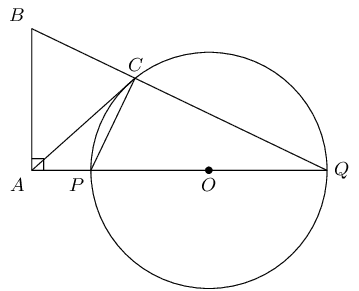$$POQ$$ is a diameter of the circle with centre $$O$$. $$QP$$ is protruded to $$A$$ and $$AC$$ is a tangent to the circle. $$BA \perp AQ$$ and $$BCQ$$ is a straight line. Prove:

$$P\hat{C}Q = B\hat{A}P$$
$\begin{array}{rll} P\hat{C}Q &= \text{90}\text{°} & (\angle \text{ in semi-circle } ) \\ B\hat{A}Q &= \text{90}\text{°} & ( \text{ given } BA \perp AQ) \\ \therefore P\hat{C}Q &= B\hat{A}Q & \end{array}$

$$BAPC$$ is a cyclic quadrilateral

$\begin{array}{rll} P\hat{C}Q &= B\hat{A}Q & ( \text{ proven } ) \\ \therefore &BAPC \text{ is a cyclic quad. } & ( \text{ ext. angle = int. opp. angle } ) \end{array}$
$$AB = AC$$
$\begin{array}{rll} C\hat{P}Q &= A\hat{B}C & ( \text{ ext. angle of cyclic quad. } ) \\ B\hat{C}P &= C\hat{P}Q + C\hat{Q}P & ( \text{ ext. angle of } \triangle ) \\ A\hat{C}P &= C\hat{Q}P & ( \text{ tangent-chord } ) \\ \therefore B\hat{C}A &= C\hat{P}Q & \\ \therefore &= A\hat{B}C & \\ \therefore AB &= AC & \text{ angles opp. equal sides} \end{array}$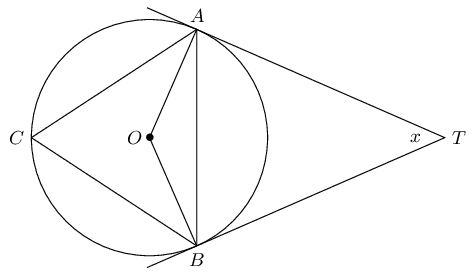$$TA$$ and $$TB$$ are tangents to the circle with centre $$O$$. $$C$$ is a point on the circumference and $$A\hat{T}B = x$$. Express the following in terms of $$x$$, giving reasons:

$$A\hat{B}T$$
$$A\hat{B}T = \text{90}\text{°} - \frac{x}{2}$$
$$O\hat{B}A$$
$$O\hat{B}A = \frac{x}{2}$$
$$\hat{C}$$
$$\hat{C} = \text{90}\text{°} - \frac{x}{2}$$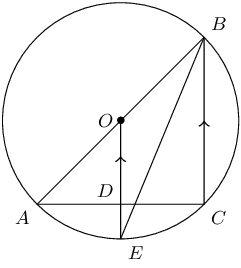$$AOB$$ is a diameter of the circle $$AECB$$ with centre $$O$$. $$OE \parallel BC$$ and cuts $$AC$$ at $$D$$.

Prove $$AD = DC$$

$\begin{array}{rll} BC &\parallel OE & ( \text{ given } ) \\ A\hat{C}B &= \text{90}\text{°} & (\angle \text{ in semi-circle } ) \\ \therefore O\hat{D}C &= \text{90}\text{°} & ( \text{ corresp. } \angle, BC \parallel OE ) \\ \therefore AD &= DC & ( \text{ perp. from centre to mid-point. } ) \end{array}$

Show that $$A\hat{B}C$$ is bisected by $$EB$$

$\begin{array}{rll} O\hat{E}B &= E\hat{B}C & ( \text{ alt. } \angle, OE \parallel BC ) \\ OE &= OB & (\angle \text{ equal radii} ) \\ \therefore O\hat{E}B &= O\hat{B}E & (\angle \text{ opp. equal sides } ) \\ \therefore O\hat{B}E &= E\hat{B}C & \\ \therefore A\hat{B}C &\text{ is bisected} & \end{array}$

If $$O\hat{E}B = x$$, express $$B\hat{A}C$$ in terms of $$x$$

$\begin{array}{rll} \text{In } &\triangle BAC, & \\ B\hat{A}C &= \text{180}\text{°} - \text{90}\text{°} -2x & (\angle \text{ sum of } \triangle ) \\ \therefore B\hat{A}C &= \text{90}\text{°} -2x & \end{array}$

Calculate the radius of the circle if $$AC = \text{10}\text{ cm}$$ and $$DE = \text{1}\text{ cm}$$

$\begin{array}{rll} \text{In } &\triangle AOD, & \\ \text{Let } AO &=r & \\ AO^2 &= 5^2 + (r-1)^2 & (\text{ Pythagoras }) \\ r^2 &= 25 + r^2 - 2r + 1 & \\ 2r &= 26 & \\ \therefore r &= \text{13}\text{ cm} & \end{array}$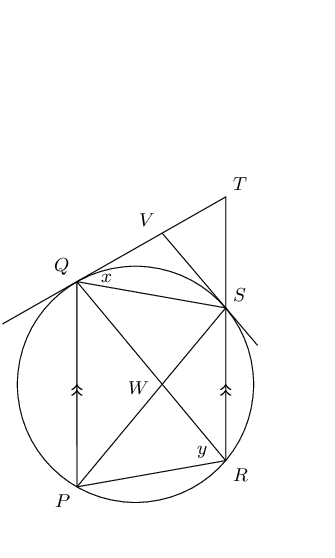$$PQ$$ and $$RS$$ are chords of the circle and $$PQ \parallel RS$$. The tangent to the circle at $$Q$$ meets $$RS$$ protruded at $$T$$. The tangent at $$S$$ meets $$QT$$ at $$V$$. $$QS$$ and $$PR$$ are drawn.

Let $$T\hat{Q}S = x$$ and $$Q\hat{R}P = y$$. Prove that:

$$T\hat{V}S = 2 Q\hat{R}S$$
$\begin{array}{rll} \text{In } &\triangle VQS, & \\ VQ &= VS & ( \text{ tangents from same pt. } ) \\ \therefore V\hat{S}Q &= V\hat{Q}S = x & (\angle \text{s opp. equal sides }) \\ \therefore T\hat{V}S &= x + x & (\text{ext. angle of } \triangle ) \\ &= 2x & \\ \text{And } Q\hat{R}S &= Q\hat{P}S = x & (\angle \text{ same seg. }) \\ \therefore T\hat{V}S &= 2Q\hat{R}S & \end{array}$

$$QVSW$$ is a cyclic quadrilateral

$\begin{array}{rll} T\hat{V}S &= 2Q\hat{R}S & ( \text{ proven } )\\ V\hat{Q}S &= Q\hat{P}S & ( \text{ tangent-chord } )\\ Q\hat{P}S &= P\hat{S}R = x & ( \text{ alt. } \angle, PQ \parallel RT )\\ \text{And } Q\hat{W}S &= 2Q\hat{R}S & (\angle \text{ ext. angle of } \triangle WSR ) \\ \therefore T\hat{V}S &= Q\hat{W}S &= 2x \\ \therefore QVSW &= \text{ is cyclic quad. } & \text{ext. angle = int. opp. angle } \end{array}$
$$Q\hat{P}S + \hat{T} = P\hat{R}T$$
$\begin{array}{rll} \hat{Q} &= Q\hat{R}P = y & ( \text{ tangent-chord } )\\ \hat{Q} &= \hat{T} = y & ( \text{ alt. } \angle, PQ \parallel RT )\\ P\hat{R}T &= x + y & \\ \text{And } Q\hat{P}S &= x & (\angle \text{ proven } ) \\ \therefore Q\hat{P}S + \hat{T} &= x + y &= P\hat{R}T \end{array}$

$$W$$ is the centre of the circle

$\begin{array}{rll} Q\hat{R}S &= x & ( \text{ proven } )\\ Q\hat{W}S &= 2x & ( \text{ proven } )\\ \therefore W &= \text{ is circle centre. } & (\angle \text{ centre = 2 angle at circum. } \end{array}$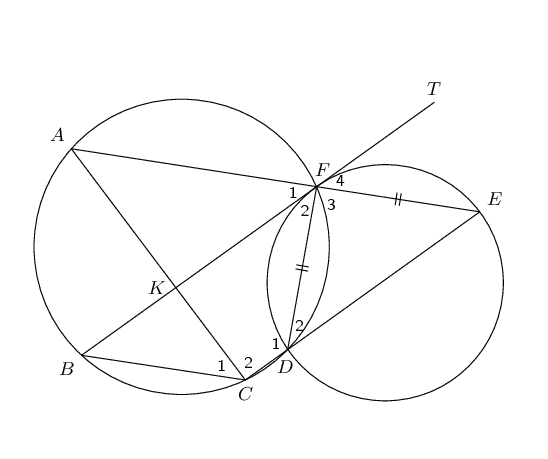The two circles shown intersect at points $$F$$ and $$D$$. $$BFT$$ is a tangent to the smaller circle at $$F$$. Straight line $$AFE$$ is drawn such that $$DF = EF$$. $$CDE$$ is a straight line and chord $$AC$$ and $$BF$$ cut at $$K$$. Prove that:

$$BT \parallel CE$$
$\begin{array}{rll} \hat{E} &= \hat{D}_2 & (\angle \text{s opp. equal sides } )\\ \hat{F}_4 &= \hat{F}_1 & ( \text{ vert. opp angles } )\\ \text{ and } \hat{F}_4 &= \hat{D}_2 & ( \text{ tangent-chord} )\\ \therefore \hat{F}_1 &= \hat{D}_2 & \\ \therefore BT &\parallel CE & (\angle \text{ corresp. angles } \end{array}$

$$BCEF$$ is a parallelogram

$\begin{array}{rll} \therefore BT & \parallel CE & ( \text{ proven}) \\ \therefore \hat{F}_1 &= \hat{C}_1 & ( \text{ angles same seg.}) \\ \therefore AE &\parallel BC & (\angle \text{ alt. angles } \\ \therefore BCEF &\text{ is parallelogram } & (\angle \text{ both opp. sides } \parallel \end{array}$
$$AC = BF$$
$\begin{array}{rll} \hat{F}_1 &= \hat{C}_1 & ( \text{ proven }) \\ \hat{F}_1 &= \hat{E} & ( \text{ proven }) \\ \hat{A} &= \hat{C}_1 & ( \text{ alt. angles }, AE \parallel BC ) \\ \therefore \hat{A} &= \hat{E} & \\ \therefore AC &= CE & (\angle \text{ angles opp. equal sides } \\ BF &= CE & (\angle \text{ opp. sides parm. =}) \\ \therefore AC &= BF & \end{array}$